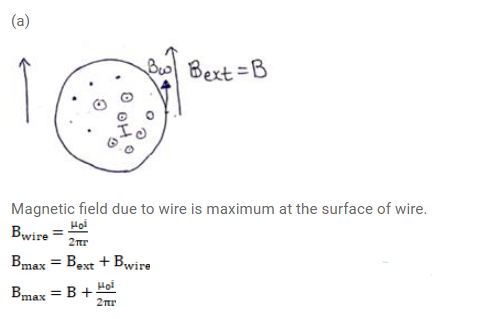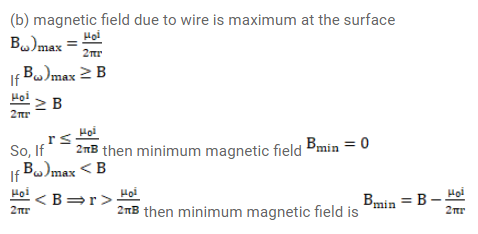# A long, straight wire of radius r carries a current i and

Question:

A long, straight wire of radius $r$ carries a current $i$ and placed horizontally in a uniform magnetic field B pointing vertically upward. The current is uniformly distributed over its cross-section.

(a) At what points will the resultant magnetic field have maximum magnitude?

(b) What will be the minimum magnitude of the resultant magnetic field?

Solution: